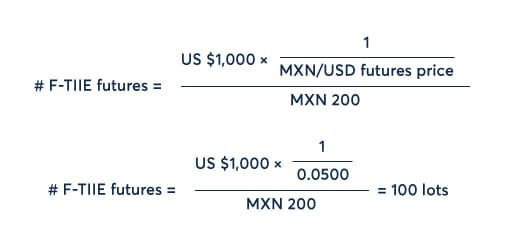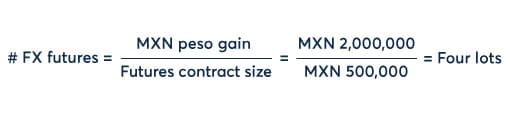# Mexican F-TIIE Futures and FX Hedging

• 7 Jul 2021
• By CME Group

The newly launched Mexican F-TIIE Interest Rate futures from CME Group provide a risk management tool for overnight Mexican peso interest rates. This paper examines cross-currency interest rate trading strategies using the F-TIIE futures contract and the opportunities for hedging the FX exposure created using CME’s MXN/USD Foreign Exchange futures contract.

In this paper, we will look at trading the interest rate differential between Mexican peso and US dollar interest rates using F-TIIE and 1-Month SOFR Interest Rate futures. Trading this differential will, in practice, likely mean trading a strip of F-TIIE futures against a strip of 1-Month SOFR futures. For example, if the expectation is for Mexican interest rates to rise faster or sooner than US interest rates, then selling the F-TIIE futures strip and buying the SOFR futures strip would meet this requirement. However, in order to highlight the FX component, we will simplify the transaction to look at a spread position for a single futures contract month.

The futures spread is created to benefit from a widening of the differential between the two interest rates. As both futures contracts are quoted in terms of “100 – Rate,” this means the futures position is created by selling the F-TIIE futures contract and buying the 1-Month SOFR futures contract.

Let’s take an example where a trader is taking on this position with exposure of \$1000 per basis point. To achieve this with 1-Month SOFR futures, which have a basis point value of \$41.67, a position of 24 lots is required:To determine the equivalent position for F-TIIE futures, the Mexican peso/US dollar exchange rate is required in order to convert the \$1000 basis point value to a Mexican peso value. The price of a CME Mexican Peso FX futures contract can be used to do this. Prices for the Mexican Peso futures are quoted in terms of US dollars per peso. Let’s establish a starting position with a futures price of US\$ 0.0500 per peso, which is equivalent to an FX rate of 20 pesos per dollar. The basis point value of the F-TIIE futures is 200 Mexican pesos. The number of F-TIIE futures required can be calculated as follows:Our spread position is therefore long 24 1-Month SOFR futures, short 100 F-TIIE futures. The interest rate differential that has been locked in is 4.50%.

At this point, the FX exposure of this position is zero – the exposure is limited to movements in the two interest rates. As the number of F-TIIE futures has been calculated using the FX rate, should the FX rate change, this will affect the hedge ratio and F-TIIE futures may need to be bought or sold in order to maintain equivalent dollar exposure.

Initial Transaction

Contract

Position

Price

1-Month SOFR futures

Long 24 lots

99.92

F-TIIE futures

Short 100 lots

95.42

An FX exposure will arise should the interest rates, and therefore, the futures prices change.

Let’s imagine that interest rates change in both currencies. 1-Month SOFR increases 0.50% and F-TIIE increases 1.00%. The rate differential has widened, and therefore, the spread trade should yield a positive return. 1-Month SOFR futures prices have fallen 50 basis points – with each basis point valued at \$41.67 and 24 lots held, this equates to a loss of US \$50K. F-TIIE futures prices have fallen 100 basis points – each basis point is valued at MXN 200 and with 100 lots held, the gain on this leg of the trade equates to MXN 2M. Assuming the FX rate is unchanged, this is equal to a gain of US \$100K. For the strategy as a whole, the net gain is US \$50K or MXN 1M ‒ depending on your base currency.

Transaction Value

Contract

Position

Price

Gain (+) / Loss (-)

US\$ Gain/Loss

MXN Gain/Loss

1-Month SOFR Futures

Long 24 lots

99.42

- US\$ 50,004

- US\$ 50,004

- MXN 1,000,080

F-TIIE Futures

Short 100 lots

94.42

+ MXN 2,000,000

+ US\$ 100,000

+ MXN 2,000,000

This position now has an FX exposure. Assuming the interest rate spread position is kept open, the FX risk can be hedged with CME Mexican Peso futures.

Whilst the net gain on the spread trade is US \$50K, the structure of the FX hedge depends on two different data points. Firstly, the hedge requirement will depend on the base currency of the trader.  If the base currency is US dollars, then the gain made on the F-TIIE futures measured in pesos contains the FX risk. The loss on the 1-Month SOFR futures is measured in US dollars, and therefore, has no FX risk for the trader. If, however, the trader’s base currency is Mexican peso, then the loss made on the 1-Month SOFR position contains the FX risk.

Secondly, the hedge requirement will depend on whether the exposure that creates the FX risk is a gain or a loss. For example, an increase in the USD/MXN FX rate (i.e. the number of Mexican pesos per US dollar) will increase the MXN value of a US dollar gain but will also increase the MXN value of a US dollar loss. Each of these outcomes has a different desirability and each would require a different hedging strategy.

In our example, let’s first take a trader with the US dollar as a base currency. The FX exposure relates to the MXN 2M gain on the F-TIIE futures. The prevailing FX rate, as observed in the MXN/USD FX futures price, is 0.0500 US dollars per Mexican peso. If this value were to fall, the dollar value of the gain would be less. This outcome could be hedged by selling FX futures. Each MXN/USD FX futures contract from CME has a size of MXN 500K. Therefore, hedging the position requires four FX futures.Alternatively, let’s look at a trader with the Mexican peso as a base currency. The FX exposure relates to the US \$50K loss made on the 1-Month SOFR futures. If the prevailing FX rate of 0.0500 US dollars per Mexican peso were to fall, this loss as measured in pesos would increase. This outcome could be hedged by selling FX futures. In this case, hedging the position would require two FX futures.We can see what happens when the FX rate falls to 0.0480 (equivalent to MXN 20.833 per US dollar).

For the US dollar-based trader, the fall in the MXN/USD rate has reduced the value of F-TIIE futures gain by US \$4K. To compensate, the short position in MXN/USD futures has gained US \$4K.

Transaction Value

Contract

Position

Price

Gain (+) / Loss (-)

US\$ Gain/Loss

FX Impact

1-Month SOFR Futures

Long 24 lots

99.42

- US\$ 50,004

- US\$ 50,004

+ US\$ 0

F-TIIE Futures

Short 100 lots

94.42

+ MXN 2,000,000

+ US\$ 96,000

- US\$ 4,000

MXN/USD Futures

Short 4 lots

0.0480

+ US\$ 4,000

+ US\$ 4,000

+ US\$ 4,000

For the Mexican peso-based trader, the fall in the MXN/USD rate has increased the peso value of the loss on the 1-Month SOFR futures by MXN 41,670. At the updated prevailing exchange rate, the US \$2,000 gain on the short FX futures position equates to a gain of MXN 41,667 ‒ effectively offsetting the additional loss on the SOFR position.

Transaction Value

Contract

Position

Price

Gain (+) / Loss (-)

MXN Gain/Loss

FX Impact

1-Month SOFR Futures

Long 24 lots

99.42

- US\$ 50,004

- MXN 1,041,750

- MXN 41,670

F-TIIE Futures

Short 100 lots

94.42

+ MXN 2,000,000

+ MXN 2,000,000

+ MXN 0

MXN/USD Futures

Short two lots

0.0480

+ US\$ 2,000

+ MXN 41,667

+ MXN 41,667

CME MXN/USD futures are an effective hedging tool for the FX risk that is present in cross-currency interest rate spread trades. The hedging requirement depends on the size and direction of the gains and losses on interest rate futures and the base currency of the trader. As this position is dynamic, the FX hedging requirement should be re-assessed frequently.